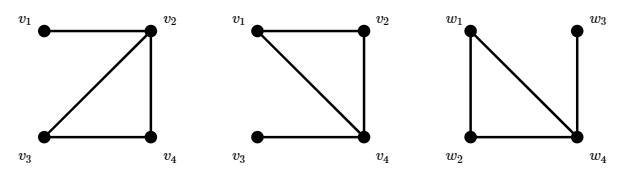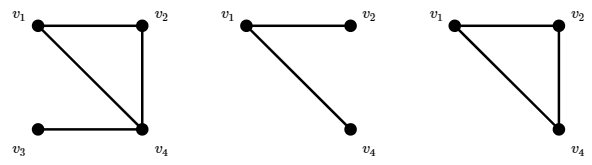$$\newcommand{\id}{\mathrm{id}}$$ $$\newcommand{\Span}{\mathrm{span}}$$ $$\newcommand{\kernel}{\mathrm{null}\,}$$ $$\newcommand{\range}{\mathrm{range}\,}$$ $$\newcommand{\RealPart}{\mathrm{Re}}$$ $$\newcommand{\ImaginaryPart}{\mathrm{Im}}$$ $$\newcommand{\Argument}{\mathrm{Arg}}$$ $$\newcommand{\norm}{\| #1 \|}$$ $$\newcommand{\inner}{\langle #1, #2 \rangle}$$ $$\newcommand{\Span}{\mathrm{span}}$$

# 5.1: The Basics of Graph Theory

$$\newcommand{\vecs}{\overset { \rightharpoonup} {\mathbf{#1}} }$$ $$\newcommand{\vecd}{\overset{-\!-\!\rightharpoonup}{\vphantom{a}\smash {#1}}}$$$$\newcommand{\id}{\mathrm{id}}$$ $$\newcommand{\Span}{\mathrm{span}}$$ $$\newcommand{\kernel}{\mathrm{null}\,}$$ $$\newcommand{\range}{\mathrm{range}\,}$$ $$\newcommand{\RealPart}{\mathrm{Re}}$$ $$\newcommand{\ImaginaryPart}{\mathrm{Im}}$$ $$\newcommand{\Argument}{\mathrm{Arg}}$$ $$\newcommand{\norm}{\| #1 \|}$$ $$\newcommand{\inner}{\langle #1, #2 \rangle}$$ $$\newcommand{\Span}{\mathrm{span}}$$ $$\newcommand{\id}{\mathrm{id}}$$ $$\newcommand{\Span}{\mathrm{span}}$$ $$\newcommand{\kernel}{\mathrm{null}\,}$$ $$\newcommand{\range}{\mathrm{range}\,}$$ $$\newcommand{\RealPart}{\mathrm{Re}}$$ $$\newcommand{\ImaginaryPart}{\mathrm{Im}}$$ $$\newcommand{\Argument}{\mathrm{Arg}}$$ $$\newcommand{\norm}{\| #1 \|}$$ $$\newcommand{\inner}{\langle #1, #2 \rangle}$$ $$\newcommand{\Span}{\mathrm{span}}$$

See Section 4.5 to review some basic terminology about graphs.

A graph $$G$$ consists of a pair $$(V,E)$$, where $$V$$ is the set of vertices and $$E$$ the set of edges. We write $$V(G)$$ for the vertices of $$G$$ and $$E(G)$$ for the edges of $$G$$ when necessary to avoid ambiguity, as when more than one graph is under discussion.

If no two edges have the same endpoints we say there are no multiple edges, and if no edge has a single vertex as both endpoints we say there are no loops. A graph with no loops and no multiple edges is a simple graph. A graph with no loops, but possibly with multiple edges is a multigraph. The condensation of a multigraph is the simple graph formed by eliminating multiple edges, that is, removing all but one of the edges with the same endpoints. To form the condensation of a graph, all loops are also removed. We sometimes refer to a graph as a general graph to emphasize that the graph may have loops or multiple edges.

The edges of a simple graph can be represented as a set of two element sets; for example, $(\{v_1,\ldots,v_7\},\{\{v_1,v_2\},\{v_2,v_3\},\{v_3,v_4\},\{v_3,v_5\}, \{v_4,v_5\},\{v_5,v_6\},\{v_6,v_7\}\})\nonumber$ is a graph that can be pictured as in Figure $$\PageIndex{1}$$. This graph is also a connected graph: each pair of vertices $$v$$, $$w$$ is connected by a sequence of vertices and edges, $$v=v_1,e_1,v_2,e_2,\ldots,v_k=w$$, where $$v_i$$ and $$v_{i+1}$$ are the endpoints of edge $$e_{i}$$. The graphs shown in Figure 4.5.2 are connected, but the figure could be interpreted as a single graph that is not connected.Figure $$\PageIndex{1}$$: A simple graph.

A graph $$G=(V,E)$$ that is not simple can be represented by using multisets: a loop is a multiset $$\{v,v\}=\{2\cdot v\}$$ and multiple edges are represented by making $$E$$ a multiset. The condensation of a multigraph may be formed by interpreting the multiset $$E$$ as a set.

A general graph that is not connected, has loops, and has multiple edges is shown in Figure $$\PageIndex{2}$$. The condensation of this graph is shown in Figure $$\PageIndex{3}$$.Figure $$\PageIndex{2}$$: A general graph: it is not connected and has loops and multiple edges.Figure $$\PageIndex{3}$$: The condensation of the previous graph.

The degree of a a vertex $$v$$, $$d(v)$$, is the number of times it appears as an endpoint of an edge. If there are no loops, this is the same as the number of edges incident with $$v$$, but if $$v$$ is both endpoints of an edge, namely, of a loop, then this contributes 2 to the degree of $$v$$. The degree sequence of a graph is a list of its degrees; the order does not matter, but usually we list the degrees in increasing or decreasing order. The degree sequence of the graph in Figure $$\PageIndex{2}$$, listed clockwise starting at the upper left, is $$0,4,2,3,2,8,2,4,3,2,2$$. We typically denote the degrees of the vertices of a graph by $$d_i$$, $$i=1,2,\ldots,n$$, where $$n$$ is the number of vertices. Depending on context, the subscript $$i$$ may match the subscript on a vertex, so that $$d_i$$ is the degree of $$v_i$$, or the subscript may indicate the position of $$d_i$$ in an increasing or decreasing list of the degrees; for example, we may state that the degree sequence is $$d_1\le d_2\le \cdots\le d_n$$.

Our first result, simple but useful, concerns the degree sequence.

Theorem $$\PageIndex{1}$$

In any graph, the sum of the degree sequence is equal to twice the number of edges, that is, $\sum_{i=1}^n d_i = 2|E|.\nonumber$

Proof

Let $$d_i$$ be the degree of $$v_i$$. The degree $$d_i$$ counts the number of times $$v_i$$ appears as an endpoint of an edge. Since each edge has two endpoints, the sum $$\sum_{i=1}^n d_i$$ counts each edge twice.

An easy consequence of this theorem:

Corollary $$\PageIndex{1}$$

The number of odd numbers in a degree sequence is even.

An interesting question immediately arises: given a finite sequence of integers, is it the degree sequence of a graph? Clearly, if the sum of the sequence is odd, the answer is no. If the sum is even, it is not too hard to see that the answer is yes, provided we allow loops and multiple edges. The sequence need not be the degree sequence of a simple graph; for example, it is not hard to see that no simple graph has degree sequence $$0,1,2,3,4$$. A sequence that is the degree sequence of a simple graph is said to be graphical. Graphical sequences have be characterized; the most well known characterization is given by this result:

Theorem $$\PageIndex{2}$$

A sequence $$d_1\ge d_2\ge \ldots\ge d_n$$ is graphical if and only if $$\sum_{i=1}^n d_i$$ is even and for all $$k\in \{1,2,\ldots,n\}$$, $\sum_{i=1}^k d_i\le k(k-1)+\sum_{i=k+1}^n \min(d_i,k).\nonumber$

It is not hard to see that if a sequence is graphical it has the property in the theorem; it is rather more difficult to see that any sequence with the property is graphical.

What does it mean for two graphs to be the same? Consider these three graphs: \eqalign{ G_1&=(\{v_1,v_2,v_3,v_4\},\{\{v_1,v_2\},\{v_2,v_3\},\{v_3,v_4\},\{v_2,v_4\}\})\cr G_2&=(\{v_1,v_2,v_3,v_4\},\{\{v_1,v_2\},\{v_1,v_4\},\{v_3,v_4\},\{v_2,v_4\}\})\cr G_3&=(\{w_1,w_2,w_3,w_4\},\{\{w_1,w_2\},\{w_1,w_4\},\{w_3,w_4\},\{w_2,w_4\}\})\cr }\nonumber These are pictured in Figure $$\PageIndex{4}$$. Simply looking at the lists of vertices and edges, they don't appear to be the same. Looking more closely, $$G_2$$ and $$G_3$$ are the same except for the names used for the vertices: $$v_i$$ in one case, $$w_i$$ in the other. Looking at the pictures, there is an obvious sense in which all three are the same: each is a triangle with an edge (and vertex) dangling from one of the three vertices. Although $$G_1$$ and $$G_2$$ use the same names for the vertices, they apply to different vertices in the graph: in $$G_1$$ the "dangling'' vertex (officially called a pendant vertex) is called $$v_1$$, while in $$G_2$$ it is called $$v_3$$. Finally, note that in the figure, $$G_2$$ and $$G_3$$ look different, even though they are clearly the same based on the vertex and edge lists.Figure $$\PageIndex{4}$$: Three isomorphic graphs.

So how should we define "sameness'' for graphs? We use a familiar term and definition: isomorphism.

Definition $$\PageIndex{1}$$: Isomorphic & Isomorphism

Suppose $$G_1=(V,E)$$ and $$G_2=(W,F)$$. $$G_1$$ and $$G_2$$ are isomorphic if there is a bijection $$f\colon V\to W$$ such that $$\{v_1,v_2\}\in E$$ if and only if $$\{f(v_1),f(v_2)\}\in F$$. In addition, the repetition numbers of $$\{v_1,v_2\}$$ and $$\{f(v_1),f(v_2)\}$$ are the same if multiple edges or loops are allowed. This bijection $$f$$ is called an isomorphism. When $$G_1$$ and $$G_2$$ are isomorphic, we write $$G_1\cong G_2$$.

Each pair of graphs in Figure $$\PageIndex{4}$$ are isomorphic. For example, to show explicitly that $$G_1\cong G_3$$, an isomorphism is \eqalign{ f(v_1)&=w_3\cr f(v_2)&=w_4\cr f(v_3)&=w_2\cr f(v_4)&=w_1.\cr }\nonumber

Clearly, if two graphs are isomorphic, their degree sequences are the same. The converse is not true; the graphs in Figure $$\PageIndex{5}$$ both have degree sequence $$1,1,1,2,2,3$$, but in one the degree-2 vertices are adjacent to each other, while in the other they are not. In general, if two graphs are isomorphic, they share all "graph theoretic'' properties, that is, properties that depend only on the graph. As an example of a non-graph theoretic property, consider "the number of times edges cross when the graph is drawn in the plane.''Figure $$\PageIndex{5}$$: Non-isomorphic graphs with degree sequence $$1,1,1,2,2,3$$.

In a more or less obvious way, some graphs are contained in others.

Definition $$\PageIndex{2}$$: Subgraph & Induced Subgraph

Graph $$H=(W,F)$$ is a subgraph of graph $$G=(V,E)$$ if $$W\subseteq V$$ and $$F\subseteq E$$. (Since $$H$$ is a graph, the edges in $$F$$ have their endpoints in $$W$$.) $$H$$ is an induced subgraph if $$F$$ consists of all edges in $$E$$ with endpoints in $$W$$. See Figure $$\PageIndex{6}$$. Whenever $$U\subseteq V$$ we denote the induced subgraph of $$G$$ on vertices $$U$$ as $$G[U]$$.Figure $$\PageIndex{6}$$: Left to right: a graph, a subgraph, an induced subgraph.

A path in a graph is a subgraph that is a path; if the endpoints of the path are $$v$$ and $$w$$ we say it is a path from $$v$$ to $$w$$. A cycle in a graph is a subgraph that is a cycle. A clique in a graph is a subgraph that is a complete graph.

If a graph $$G$$ is not connected, define $$v\sim w$$ if and only if there is a path connecting $$v$$ and $$w$$. It is not hard to see that this is an equivalence relation. Each equivalence class corresponds to an induced subgraph $$G$$; these subgraphs are called the connected components of the graph.